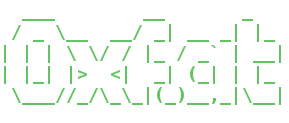# Level 19The following password has been encrypted by the telephone alphabet pattern. The solution for this level is the digit sum of the number code

Example:
A => 22
B => 222
C => 2222
1 => 1
2 => 2
5 => 5
I => 4444
W => 99
Z => 99999

"HEY" => 444 333 9999 => 4+4+4+3+3+3+9+9+9+9 = 57

``ae7a7922482f69c22cbf6a6508cab0e9``